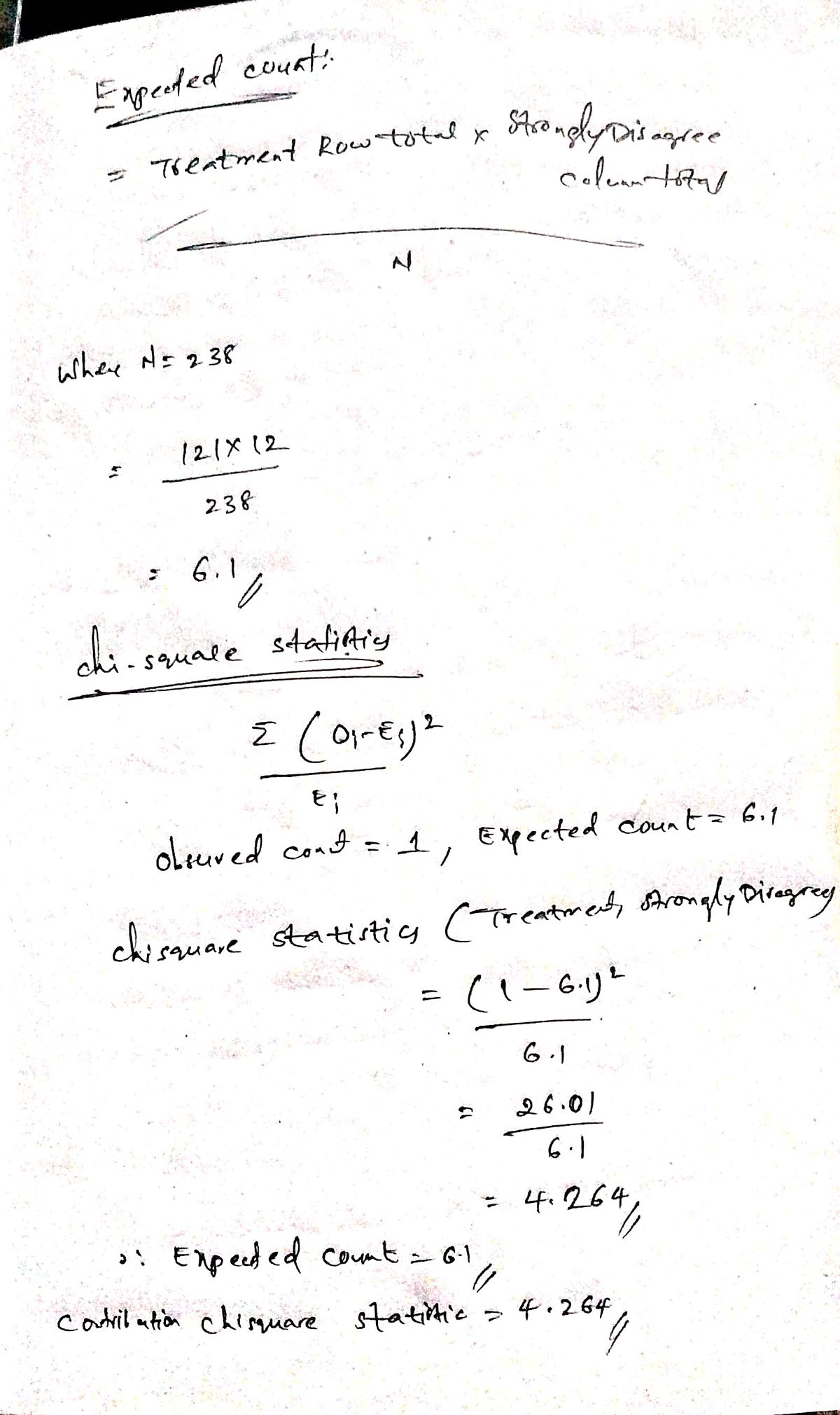Leah Vasquez

2022-06-19

Find the expected count and the contribution to the chi-square statistic for the (Group 1, No) cell in the two-way table below.

Round your answer for the expected count to one decimal place, and your answer for the contribution to the chi-square statistic to three decimal places.

Find the expected count and the contribution to the chi-square statistic for the (C,D)

cell in the two-way table below.

Round your answer for the expected count to one decimal place, and your answer for the contribution to the chi-square statistic to three decimal places.

Find the expected count and the contribution to the chi-square statistic for the (Treatment, Strongly Disagree) cell in the two-way table below.

Round your answer for the excepted count to one decimal place, and your answer for the contribution to the chi-square statistic to three decimal places.

Find the expected count and the contribution to the chi-square statistic for the (Group 1, No) cell in the two-way table below.

Round your answer for the excepted count to one decimal place, and your answer for the contribution to the chi-square statistic to three decimal places.

Give the degrees of freedom for the chi-square test based on the two-way table.

Consider the data with analysis shown in the following computer output:

What is the pooled standard deviation? What degrees of freedom are used in doing inferences for these means and differences in means?

Round your answer for the pooled standard deviation to two decimal places.

Consider the data with analysis shown in the following computer output:

What is the pooled standard deviation? What degrees of freedom are used in doing inferences for these means and differences in means?

Round your answer for the pooled standard deviation to two decimal places

Use the following ANOVA table for regression to answer the questions.

Analysis of Variance

Give the F-statistic and p-value.

Use the following ANOVA table for regression to answer the questions.

Analysis of Variance

Give the F-statistic and p-value.

Use the following ANOVA table for regression to answer the questions.

Response: Y

Give the F-statistic and p-value.

Use the following ANOVA table for regression to answer the questions.

Response: Y

Give the F-statistic and p-value.

Two intervals are given, A and B, for the same value of the explanatory variable.

(a) Which interval is the confidence interval for the mean response?

Choose the answer from the menu in accordance to item (a) of the question statement

Which interval is the prediction interval for the response?

Choose the answer from the menu in accordance to item (a) of the question statement

(b) What is the predicted value of the response variable for this value of the explanatory variable?

Two intervals are given, A and B, for the same value of the explanatory variable.

(a) Which interval is the confidence interval for the mean response?

Choose the answer from the menu in accordance to item (a) of the question statement

Which interval is the prediction interval for the response?

Choose the answer from the menu in accordance to item (a) of the question statement

(b) What is the predicted value of the response variable for this value of the explanatory variable?

Two intervals are given, A and B, for the same value of the explanatory variable.

(a) Which interval is the confidence interval for the mean response?

Choose the answer from the menu in accordance to item (a) of the question statement

Which interval is the prediction interval for the response?

Choose the answer from the menu in accordance to item (a) of the question statement

(b) What is the predicted value of the response variable for this value of the explanatory variable?

Two intervals are given, A and B, for the same value of the explanatory variable.

(a) Which interval is the confidence interval for the mean response?

Choose the answer from the menu in accordance to item (a) of the question statement

Which interval is the prediction interval for the response?

Choose the answer from the menu in accordance to item (a) of the question statement

(b) What is the predicted value of the response variable for this value of the explanatory variable?Expert

Q2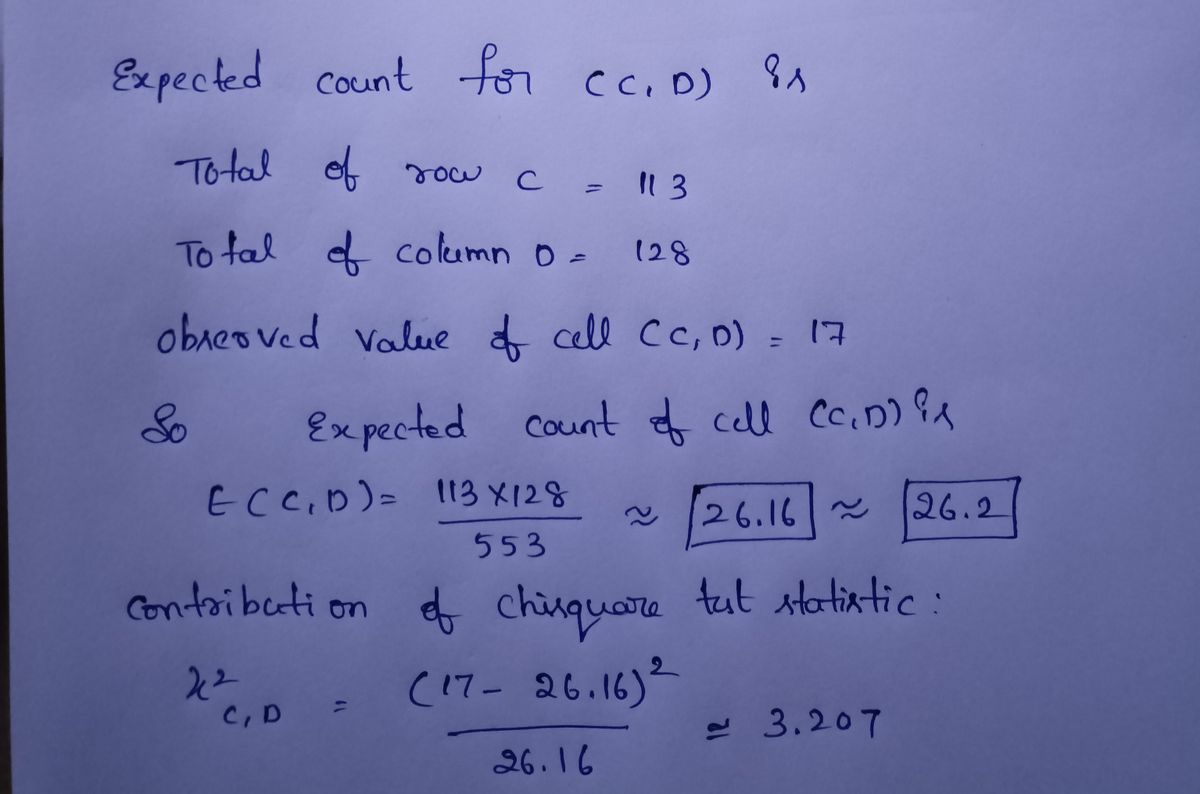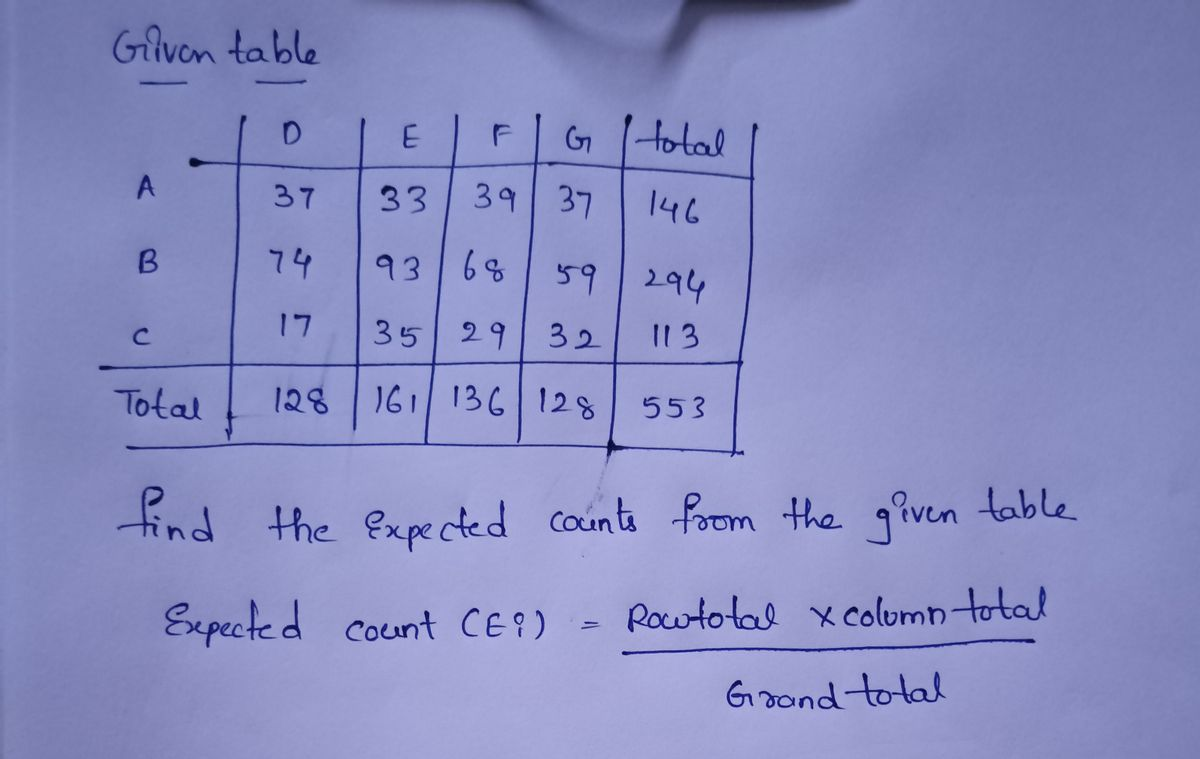Expert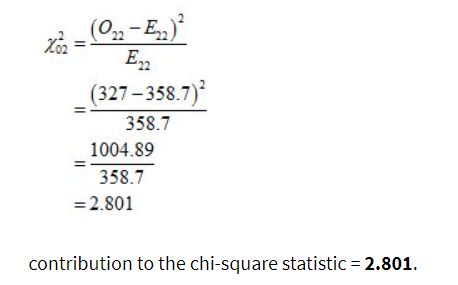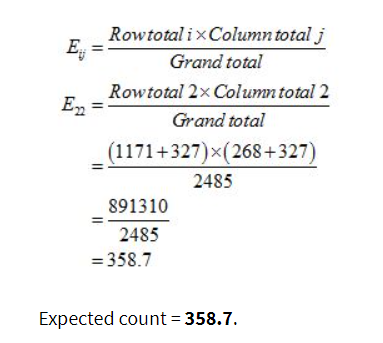Q4.There are two categorical variables which are group and opinion.

We have to find the expected count and the contribution to the chi-square statistic for the (Group 2, No) cell.

This is Chi-Square test for independence.Expert

Use the following ANOVA table for regression to answer the questions.

Response: Y

Give the F-statistic and p-value.

Use the following ANOVA table for regression to answer the questions.

Response: Y

Give the F-statistic and p-value.Expert

Use the following ANOVA table for regression to answer the questions.

Analysis of Variance

Give the F-statistic and p-value.Expert

What is the pooled standard deviation? What degrees of freedom are used in doing inferences for these means and differences in means?
Round your answer for the pooled standard deviation to two decimal places.

Consider the data with analysis shown in the following computer output:Expert

Q1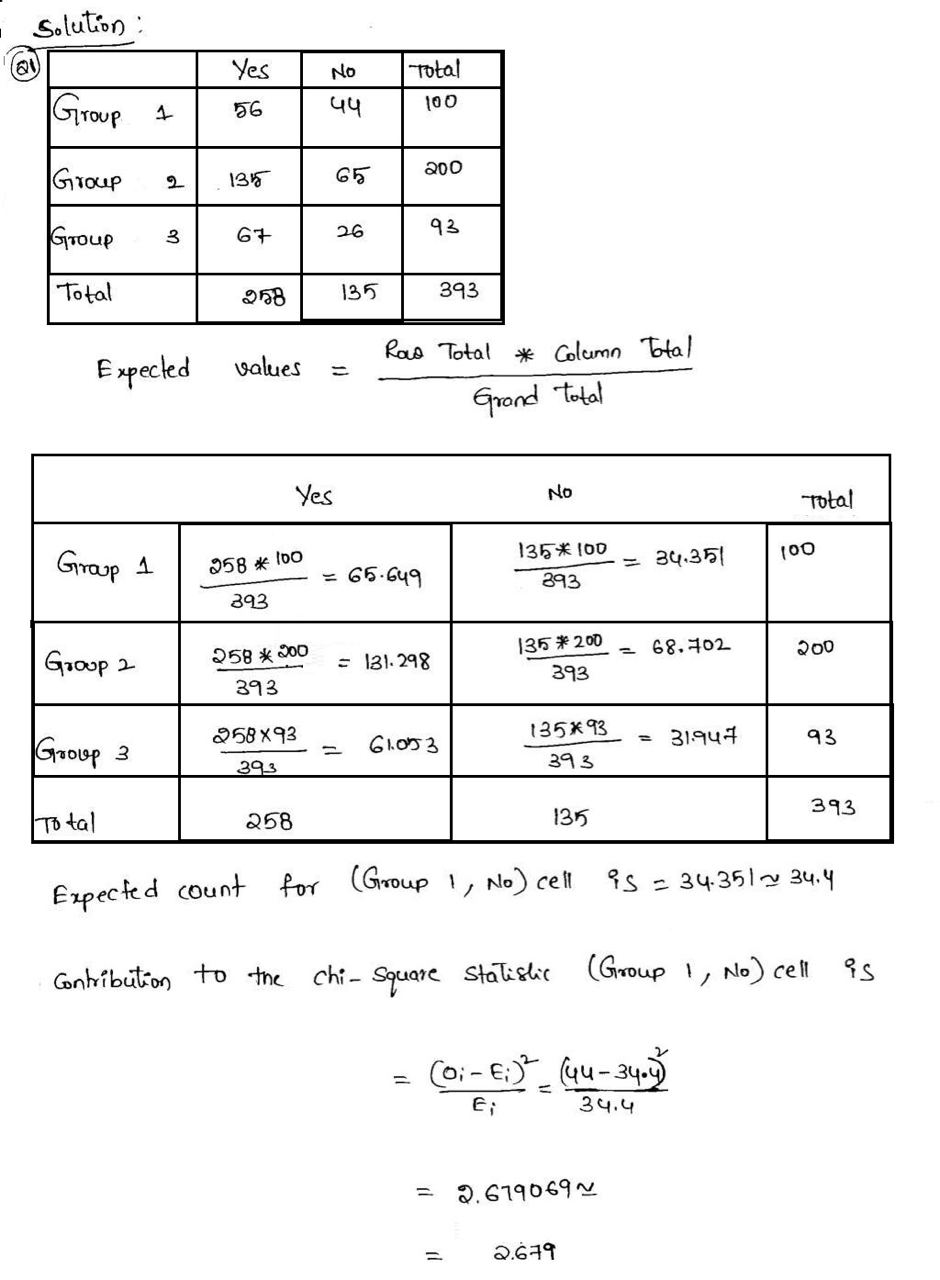Expert

Round your answer for the pooled standard deviation to two decimal places

Use the following ANOVA table for regression to answer the questions.

Analysis of Variance

Give the F-statistic and p-value.

It is given that

Mean sum of square of error is MSE = 154.9

The pooled standard deviation can be calculated as

Pooled SD = √(MSE) = √(154.9) = 12.45 (upto 2 decimal places)

From the given ANOVA table, the F-statistic is given by

F = 1.85

From the given ANOVA table the p-value is given by

P-value = 0.176Expert

Q3